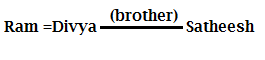# Data Sufficiency Quiz for SBI PO 2017

Direction: Each of the following questions has two statements. You have to decide whether data provided in the statements are sufficient to answer the question.

A. If the data in statement I alone are sufficient to answer the question, while the data in statement II alone is not sufficient to answer the question
B. If the data in statement II alone are sufficient to answer the question, while the data in statement I alone is not sufficient to answer the question

C. If the data either in Statement I alone or Statement II alone are sufficient to answer the question
D. I the data in both the statements I and II are not sufficient to answer the question
E. If the data in both the statement I and II are together necessary to answer the question.

1. What is the radius of the circle B?
Statement I: Diameter of circle A is 6 cm and Distance between center of circle A and B is 10 cm
Statement II: Difference between areas of both the circle is 16п

2. How is Ram related to Tarun?
Statement I: If Satheesh is only brother of Divya, who is the wife of Ram
Statement II: If Ramya is only daughter –in-law of Satheesh and Reijina is only sister of Tarun.

3. Eight Friends (A, B, C, D, E, F, G and H) are sitting in a circle facing the center. Who is sitting 3rd to the right of B?
Statement I: D and E sit opposite to each other. Immediate neighbours of D are B and G.
Statement II: E sits 3rd to the left of B, who sits 2nd to the left of G, who sits opposite of H, who sits 3rd to the right of F.

4. ‘W’ is in which direction from ‘B’?
Statement I: A is to the north of X and south of W. F is to the north of B and east of W
Statement II: C is to the south-West of W. B is to the East of C. A is to the west of B.

5. Is X>Y?
Statement I: X>Z>B; Z≥A≥W; C<D<Y
Statement II: X<Z<B; Z<W<C; B=Y>W

## Solution with Explanation:

1. Option E
From statement I:
Diameter (circle A) = 6 cm
Radius (circle A) = 6/2 = 3 cm
Distance between center of Circle A and B = 10 cm
From Statement II:
Difference between area of Circle A and B = 16 п
п (radius of circle B)² - п (radius of circle A)² =16 п
п (radius of circle B)² - п(3) ² = 16 п
п (radius of circle B)² = 16 п + 9 п = 25 п
Radius of circle B =√ 25 = 5 cm
Therefore both statements are needed to find the answer

2. Option D
From statement IFrom Statement IIThere is no direct relation between either Satheesh and Tarun or Satheesh and Reijina, so from both the statements we cannot obtain the answer.

3. Option B
From Statement I
4. Option A
From Statement I There is no relation between X and Y

From Statement II X<Z<W<Y
X<Y
Therefore Statement II alone enough to answer#### What's trending in BankExamsToday

Smart Prep Kit for Banking Exams by Ramandeep Singh - Download here